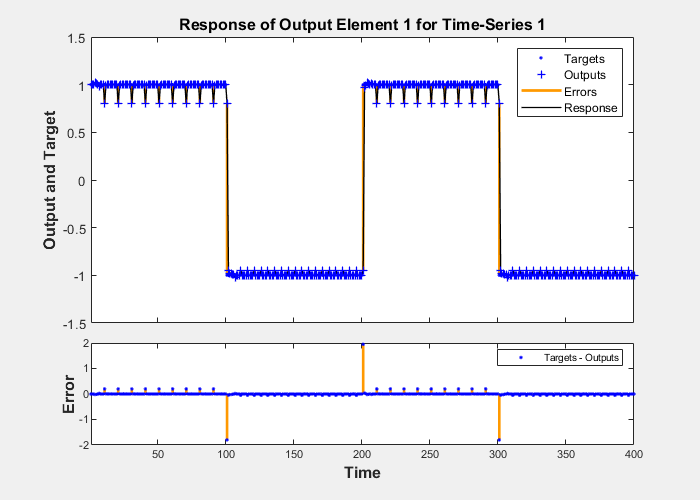## Design Time Series Distributed Delay Neural Networks

The FTDNN had the tapped delay line memory only at the input to the first layer of the static feedforward network. You can also distribute the tapped delay lines throughout the network. The distributed TDNN was first introduced in [WaHa89] for phoneme recognition. The original architecture was very specialized for that particular problem. The following figure shows a general two-layer distributed TDNN.This network can be used for a simplified problem that is similar to phoneme recognition. The network will attempt to recognize the frequency content of an input signal. The following figure shows a signal in which one of two frequencies is present at any given time.The following code creates this signal and a target network output. The target output is 1 when the input is at the low frequency and -1 when the input is at the high frequency.

```time = 0:99; y1 = sin(2*pi*time/10); y2 = sin(2*pi*time/5); y = [y1 y2 y1 y2]; t1 = ones(1,100); t2 = -ones(1,100); t = [t1 t2 t1 t2];```

Now create the distributed TDNN network with the `distdelaynet` function. The only difference between the `distdelaynet` function and the `timedelaynet` function is that the first input argument is a cell array that contains the tapped delays to be used in each layer. In the next example, delays of zero to four are used in layer 1 and zero to three are used in layer 2. (To add some variety, the training function `trainbr` is used in this example instead of the default, which is `trainlm`. You can use any training function discussed in Multilayer Shallow Neural Networks and Backpropagation Training.)

```d1 = 0:4; d2 = 0:3; p = con2seq(y); t = con2seq(t); dtdnn_net = distdelaynet({d1,d2},5); dtdnn_net.trainFcn = 'trainbr'; dtdnn_net.divideFcn = ''; dtdnn_net.trainParam.epochs = 100; dtdnn_net = train(dtdnn_net,p,t); yp = sim(dtdnn_net,p); plotresponse(t,yp)```The network is able to accurately distinguish the two “phonemes.”

You will notice that the training is generally slower for the distributed TDNN network than for the FTDNN. This is because the distributed TDNN must use dynamic backpropagation.